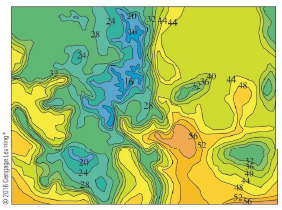Chapter 15.1, Problem 8E

Chapter
Section
Textbook Problem

The contour map shows the temperature, in degrees Fahrenheit, at 4:00 pm on February 26. 2007, in Colorado. (The state measures 388 mi west to cast and 276 mi south to north.) Use the Midpoint Rule with m = n = 4 to estimate the average temperature in Colorado at that time.To determine

To estimate: The average temperature of the Colorado state using midpoint rule.

Explanation

Formula used:

The double integral, Rf(x,y)dAi=1mj=1nf(x¯i,y¯j)ΔA ,

Where, ΔA=lb , where l, b are the length and breadth of each rectangle.

The given function is f(x,y) .

The mid points of each rectangle is denoted by (x¯i,y¯j) , where x¯i is the midpoint of [xi1,xi] and y¯i is the midpoint of [yj1,yj] .

The Riemann sum constants are denoted by m, n.

The average value of a function f of two variables defined on R is, fave=1A(R)Rf(x,y)dA , where, A(R) is the area of the given rectangle R.

Given:

The measurement of the state is [0,388]×[0,276] .

The Riemann sum constants, m=4,n=4 .

Calculation:

Plot the given rectangle in the graph and pick the mid points of each sub rectangle.

From Figure 1, it is observed that the mid points of each rectangle is,

(48.5,34.5),(48.5,103.5),(48.5,172.5),(48.5,241.5),(145.5,34.5),(145.5,103.5),(145.5,172.5),(145.5,241.5),(242.5,34.5),(242.5,103.5),(242.5,172.5),(242.5,241.5),(339.5,34.5),(339.5,103.5),(339.5,172.5),(339

Still sussing out bartleby?

Check out a sample textbook solution.

See a sample solution

The Solution to Your Study Problems

Bartleby provides explanations to thousands of textbook problems written by our experts, many with advanced degrees!

Get Started

Find more solutions based on key concepts